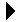Rational Number Package Spec# Rational Number Package SpecInteger Stack Demonstration Client Download Rational Number Package Body```--
-- An ADT to represent positive rational numbers.
--
package Rational_Number is
-- The type of the objects.
type Rational is private;

-- Get there from an integer or pair of integers.
function To_Rational(Num: Integer; Den: Integer := 1) return Rational;

-- Output a rational.
procedure Put(R: Rational);

-- Four functions.
function "+" (A, B: Rational) return Rational;
function "-" (A, B: Rational) return Rational;
function "*" (A, B: Rational) return Rational;
function "/" (A, B: Rational) return Rational;

-- We need to take over the equality function, otherwise 6/8 /= 3/4.
function "=" (A, B: Rational) return Boolean;

private
-- Just remember numerator and denominator.
subtype Unsigned is Integer range 0..16#7fffffff#;
type Rational is record
Num, Denom: Unsigned;
end record;
end Rational_Number;
```Integer Stack Demonstration Client Rational Number Package Body